# How do I deal with angles? Trigonometry in the geosciences

## Introduction to Trigonometry

How steep is this hillside and will it fail? How high is that mountain? These sorts of questions pop up all over in geosciences - from plate tectonics to maps to ocean waves, and they require you to find either an angle or a distance. To do this, we often use trigonometry, which is much easier when a right triangle is involved.

A right triangle (like the one in the figure to the right) has one angle that is 90°. The other two angles are always less than 90 ° and together add up to 90°. Note that the triangle on the right has 3 angles a, b and c and 3 sides, A, B, and H, and 3 angles a, b, and c. The side "opposite" an angle (in this case) is labeled with a capital letter corresponding to the label on the angle. The side opposite the right angle, H, is always the longest side and is called the hypotenuse.

## Some rules/guidelines for trigonometry of right triangles

We can use ratios (or the quotient) of the lengths of a right triangle's sides to figure out the angles in a right triangle. Three trigonometric ratios that we use in the geosciences are called the sine, the cosine, and the tangent, although they are often abbreviated sin, cos, and tan, respectively.

Because the trigonometric functions come up over and over, it is a good idea to figure out a way to remember their definitions. For most students (and, to be honest, your professors!) the mnemonic SOH-CAH-TOA (said "so-kah-toe-ahh") is helpful.

The mnemonic device uses the first letters in the following equations: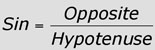(this is SOH, where S=sin, O=opposite and H=hypotenuse)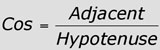(this is CAH, where c=cos, a=adjacent and H=hypotenuse)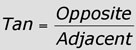(this is TOA, where t=tan, a=adjacent and O=opposite)

Because these are equations, they can be rearranged so that you can also figure out the length of a side (if you know the angle a in the example above)!

## Calculating distances and angles using trigonometry

In order to do trigonometry calculations, you should have a calculator with buttons for tan, cos, and sin. Before you begin, make sure that your calculator is in degree mode, not radian mode. If you need help with this, skip to the how do I do this on my calculator section.

In the geosciences, angles are almost always measured in degrees, where a circle is 360 degrees. Mathematicians often measure in a unit called radians. A full circle is 2π radians, since the circumference of a circle with a radius of 1 is 2π. To convert an angle measurement in radians to degrees, multiply by 57.3. To convert degrees to radians, divide by 57.3.

### How do I calculate distance?

Often when figuring out the distance to a faraway object, you know an angle and one length but need to know a second leg of a triangle. To figure out the missing side, you will substitute values into one of the trig formulas above, and solve for the missing value. For example, if you know the side adjacent to the angle and need to know the hypotenuse, you can use the formula for cosine and solve for the length you need.
Often in the geosciences we want to know the distance to a hill or other object. We can do this by triangulation. So if you measure the angle to a hill to be 135 degrees from north, and then walk 200 meters perpendicular to that and measure the angle to be 120 degrees from north, how far from the second position is the hill?
1. You will probably need to make a sketch of this problem to solve it, and to figure out which of the equations - sine, cosine or tangent you will need.
Since you walked perpendicular to the first bearing, you know you have made a right triangle and can use trigonometry to find the distance to the hill from my second position. The two bearings are 15 degrees off (135-120), thus the apex of the triangle is 15 degrees.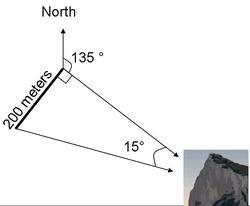2. Choose the correct formula, given the angle and side you know and which side you want.
In this question, you don't know or care about the side adjacent to the apex, so that means you will want to use the equation for the sine: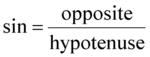3. Rearrange the equation so what you know is on one side and what you don't know is on the other, then substitute in the known values.
Since you have the angle and the opposite side, you will need to rearrange the equation so that the hypotenuse is alone: (need help with this? try the rearranging equations page)substituting in the known values we get: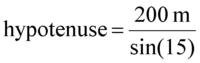4. Use your calculator to determine the value of the trigonometric function (i.e. sine, cosine or tangent) and solve for the unknown value.
Your calculator will tell you that sin(15) is 0.2588, so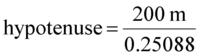therefore the hypotenuse, which is the distance to the hill is 772 meters

### How do I calculate the angle?

Often geologists need to know a gradient of a road up a hill or the angle that rocks are tilted; to calculate these angles you will need to know the lengths of two sides of triangle. From that you can determine the sine, cosine, or tangent of the angle you need.

If you have calculated the sine, cosine or tangent of the angle, and you need to know how big it is (in degrees), you will have to use the inverse functions on your calculator. (If you don't know how to use these on your calculator, jump to the how do I use my calculator? section.) For example, if you want to find the size of an angle with a sine of 0.6, you need to find inverse sine of 0.6 (written sin-1(0.6)). Try doing this on your calculator. You should get 36.87, so the angle in this case is a bit less than 37 degrees.

Sin-1 is called "inverse sine" because it does the opposite of the sine function. In the same way, the inverse cosine (cos-1) will give the value of an angle if you know its cosine and tan-1 will give you the angle if you know the tangent. In this way you can find the size of any unknown angle of a right triangle if you just know 2 sides of that triangle.

You are in charge of building a road to the top of a hill. From topographic maps, you know that the hill is 150 feet high, and the horizontal distance on the map is 1300 feet. How steep will the road be?
1. Figure out what is known, and which angle you want, and then pick the correct equation - for sine, cosine or tangent.
The hill makes a right triangle with a base of 1200 and a vertical side of 150. Since the angle in question is the lower angle opposite the vertical side, the two sides that are known are the opposite and the adjacent sides. Thus, we will use the formula for the tangent:2. Substitute the known sides into the equation you picked.
3. Use the inverse function to solve this equation for the angle that you need.
we take the inverse tangent of both sides: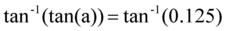Since tan-1(a) does exactly the opposite of tan(a), tan-1(tan(a)) is just a, so this becomes
a = tan-1(0.125)
which you can punch into your calculator to get the result, 7.125 degrees, the slope of the hill.

Are you ready to try some sample problems? Go on to the trig sample problem page

## How do I do this on my calculator?

Often not knowing how to use your calculator is the hardest thing about trig problems. To figure out if your calculator is in degrees or radians mode, try finding the sin(30). If your calculator gives a result of 0.5, it is in degrees mode, and if you get -.988031, it is in radian mode and you need to switch it!

To use the inverse buttons, typically you will need to press a button labeled 2nd on your calculator and then the sin, cos or tan button. This produces sin-1 on the calculator screen.

Many of the calculator companies have on-line tutorials if you need more help, including TI, Sharp, and HP.

## Why does it work?

While you may want to just "plug and chug" and use the trigonometry equations without understanding them, some folks find it helpful to understand why they work. The secret is in something called similar triangles. Similar triangles are triangles with three identical angles but different length sides such as the ones in the diagram to the right.

Similar triangles are just "blown-up" or "shrunk" (the correct term is scaled) versions of each other and so the ratio of the length of the sides remains the same. In other words the shortest side divided by the hypotenuse will always give the same result if the triangles are similar. You may already recognize that this ratio is the cosine or sine. Since similar triangles always have the same angles, then the ratio is the same for any given angle. So each ratio (whether the sin, cos or tan) has a particular angle that goes with it, and each angle has a particular ratio of sides.

## Where is trigonometry used in the geosciences?

You will find that use trigonometry in the following areas typically taught in introductory geoscience classes, plus many others:
• Mass wasting - Calculating slope steepness, gradients and forces on a hillslope, determining slope stability
• Topographic maps - determining compass direction and orienteering, calculating slopes, locating objects and landmarks, calculating the distance to objects far away
• Structural geology - measuring the tilting of rocks, determining the location of rocks in the subsurface, making geologic maps.
• Oceanography - Describing ocean waves, calculating wind and current direction
• Plate tectonics - Measuring the steepness of subduction zones
• Seismology - measuring seismic waves, determining the nature of the interior of the Earth through refraction

## Next Steps

I still need more help!
(See the links below for more help with trigonometry).

## Resources for Trigonometry

Dave's Short Trig Course has a section on angle measurement, trigonometry in right triangles, and the trigonometric functions as well as many other trigonometric topics which should be helpful.

Mathworld's Trigonometry page has a huge number or resources, that go far beyond the material typically covered in an introductory course.

S.O.S. Mathematicshas a trigonometry index , featuring a table of trigonometric identities, lessons on functions and formulae, and a section of exercises and solutions.

Math Help's trigonometry index provides a good refresher on basic trig identities, functions and angles. Also includes diagrams for unit circle and triangle trigonometry.

This page was written and compiled by Dr. Eric M. Baer, Geology Program, Highline Community College,
and Dr. Jennifer M. Wenner, Geology Department, University of Wisconsin Oshkosh